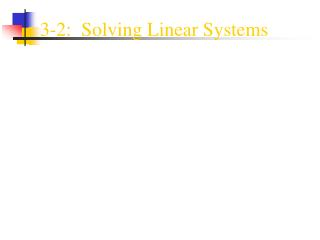Download Presentation3-2: Solving Linear Systems

# 3-2: Solving Linear Systems

Download Presentation## 3-2: Solving Linear Systems

- - - - - - - - - - - - - - - - - - - - - - - - - - - E N D - - - - - - - - - - - - - - - - - - - - - - - - - - -
##### Presentation Transcript

1. 3-2: Solving Linear Systems

2. Solving Linear Systems There are two methods of solving a system of equations algebraically: • Elimination • Substitution

3. Elimination • The key to solving a system by elimination is getting rid of one variable. • Let’s review the Additive Inverse Property. • What is the Additive Inverse of: 3x? -5y? 8p? q? -3x 5y -8p -q • What happens if we add two additive inverses? We get zero. The terms cancel. • We will try to eliminate one variable by adding, subtracting, or multiplying the variable(s) until the two terms are additive inverses. • We will then add the two equations, giving us one equation with one variable. • Solve for that variable. • Then insert the value into one of the original equations to find the other variable.

4. Elimination • Solve the system: m + n = 6 m - n = 5 • Notice that the n terms in both equations are additive inverses. So if we add the equations the n terms will cancel. • So let’s add & solve: m + n = 6 + m - n = 5 2m + 0 = 11 2m = 11 m = 11/2 or 5.5 • Insert the value of m to find n: 5.5 + n = 6 n = .5 • The solution is (5.5, .5).

5. Elimination • Solve the system: 3s - 2t = 10 4s + t = 6 We could multiply the second equation by 2 and the t terms would be inverses. OR We could multiply the first equation by 4 and the second equation by -3 to make the s terms inverses. • Let’s multiply the second equation by 2 to eliminate t. (It’s easier.) 3s - 2t = 10 3s – 2t = 10 2(4s + t = 6) 8s + 2t = 12 • Add and solve: 11s + 0t = 22 11s = 22 s = 2 • Insert the value of s to find the value of t 3(2) - 2t = 10 t = -2 • The solution is (2, -2).

6. Elimination Solve the system by elimination: 1. -4x + y = -12 4x + 2y = 6 2. 5x + 2y = 12 -6x -2y = -14 3. 5x + 4y = 12 7x - 6y = 40 4. 5m + 2n = -8 4m +3n = 2

7. Substitution • To solve a system of equations by substitution… • 1. Solve one equation for one of the variables. • 2. Substitute the value of the variable into the other • equation. • Simplify and solve the equation. • 4. Substitute back into either equation to find the value of the other variable.

8. Substitution • Solve the system: x - 2y = -5 y = x + 2 Notice: One equation is already solved for one variable. Substitute (x + 2) for y in the first equation. x - 2y = -5 x - 2(x + 2) = -5 • We now have one equation with one variable. Simplify and solve. x - 2x – 4 = -5 -x - 4 = -5 -x = -1 x = 1 • Substitute 1 for x in either equation to find y. y = x + 2 y = 1 + 2 so y = 3 • The solution is (1, 3).

9. Substitution • Let’s checkthe solution. The answer (1, 3) must check in both equations. x - 2y = -5 y = x + 2 1 - 2(3) = -5 3 = 1 + 2 -5 = -5 3 = 3 

10. Substitution • Solve the system: 2p + 3q = 2 p - 3q = -17 • Notice that neither equation is solved for a variable. Since p in the second equation does not have a coefficient, it will be easier to solve. p - 3q = -17 p = 3q – 17 • Substitute the value of p into the first equation, and solve. 2p + 3q = 2 2(3q – 17) + 3q = 2 6q – 34 + 3q = 2 9q – 34 = 2 9q = 36 q = 4

11. Substitution • Substitute the value of q into the second equation to find p. p = 3q – 17 p = 3(4) – 17 p = -5 • The solution is (-5, 4). (List p first since it comes first alphabetically.) • Let’s check the solution: 2p + 3q = 2 p – 3q = -17 2(-5) +3(4) = 2 -5 - 3(4) = -17 -10 + 12 = 2 -5 - 12 = -17 2 = 2 -17 = -17 

12. Substitution Solve the systems by substitution: 1. x = 4 2x - 3y = -19 2. 3x + y = 7 4x + 2y = 16 3. 2x + y = 5 3x – 3y = 3 4. 2x + 2y = 4 x – 2y = 0